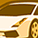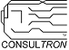# PSoC™ 5, 3 & 1 Forum DiscussionsLevel 2
Level 2# math.h functions don't work

Hello,

I am building a project where I need to know the value of the pitch from the mpu6050. For this I used following equation:
"pitch = atan2f(AX,sqrt(AY*AY + AZ*AZ))* 180/M_PI"

After this I wanted to know what the value was so I used UART to print the value to my serial monitor (used MobaXtrem), but nothing is being printed.

After a bit of testing I found out that the problem is with the math function's themself. This is because I used a simple test program in the form of:(this also didn't work)

``````float sinus;
sinus = sin(90);
// UART_PUTCHAR(sinus);
printf("the value of sin is: %f", sinus);``````

And yes I included math.h and added the 'm' in the linker.

Thank for the help

1 SolutionLevel 9
Level 9# Re: math.h functions don't work

Switten,

The problem you are having is that Creator uses the newlib-nano library by default.  This library removes the "float" conversion option in the printf-type functions to save on FLASH.  If you need the correct library to handle float values see the following KBA:  Printing-floating-point-values-in-PSoC-Creator-KBA231059

Len
"Engineering is an Art. The Art of Compromise."Level 9
Level 9# Re: math.h functions don't work